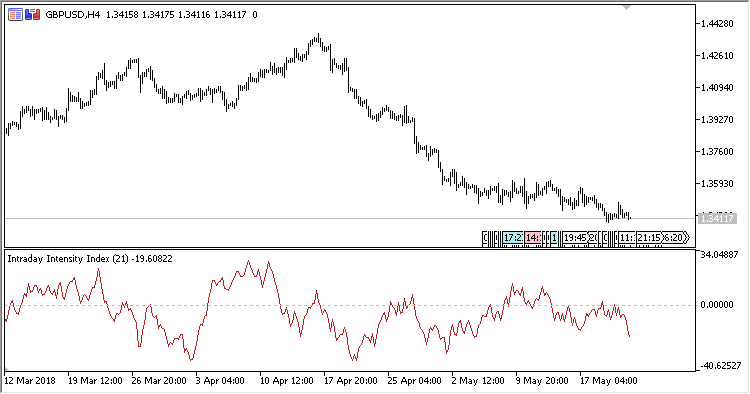# Intraday_Intensity_Index – indicator for MetaTrader 5

Intraday intensity index oscillator.

It has two input parameters:

• Period – calculation period;
• Use normalization – switch (Yes/No) of using normalization in calculations.
Related Posts

Calculations:

No normalization:

`Idx = Sum[Volume * (2*Close - High - Low) / (High - Low)] за Period`

With normalization:

`Idx = 100*(Sum[Volume * (2*Close - High - Low) / (High - Low)]) / (Sum [Volume]) за Period`